Courses

# Pavement Design Civil Engineering (CE) Notes | EduRev

## Topic wise GATE Past Year Papers for Civil Engineering

Created by: Gate Gurus

## Civil Engineering (CE) : Pavement Design Civil Engineering (CE) Notes | EduRev

The document Pavement Design Civil Engineering (CE) Notes | EduRev is a part of the Civil Engineering (CE) Course Topic wise GATE Past Year Papers for Civil Engineering.
All you need of Civil Engineering (CE) at this link: Civil Engineering (CE)

Question 1. A flexible pavement has the following class of loads during a particular hour of the day.
(i) 80 buses with 2-axles (each axle load of 40 kN);
(ii) 160 trucks with 2-axles (front and rear axle loads of 40 kN and 80 kN, respectively) The equivalent standard axle load repetitions for this vehicle combination as per IRC:37-2012 would be   [2019 : 2 Marks, Set-ll]
(a) 250
(b) 320
(c) 180
(d) 240
(c)
Solution: (i) 80 buses with 2 axle with 40 kN each.
N1 = 80 x 2 = 160
L1 = 40 kN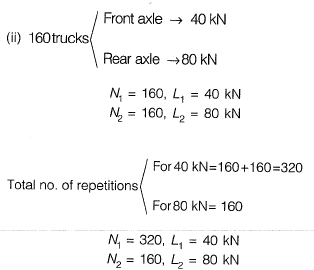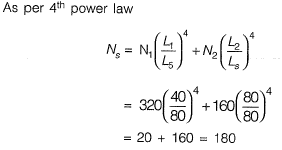Question 2. Structural failures considered in the mechanistic method of bituminous pavement design are    [2019 : 1 Mark, Set-II]
(a) Fatigue and Rutting
(b) Fatigue and Shear
(c) Shear and Slippage
(d) Rutting and Shear
(a)

Question 3. Tie bars of 12 mm diameter are to be provided in a concrete pavement slab. The working tensile stress of the tie bars is 230 MPa, the average bond strength between a tie bar and concrete is 2 MPa. and the joint gap between the slabs is 10 mm. Ignoring the loss of bond and the tolerance factor, the design length of the tie bars (in mm, round off to the nearest integer) is________.     [2019 : 2 Marks, Set-I]
Solution: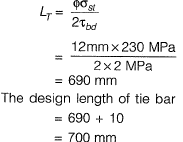Question 4.  An isolated concrete pavement slab of length L Is resting on a frictionless base. The temperature of the top and bottom fibre of the slab are Tt and Tb, respectively. Given: the coefficient of thermal expansion = α and the elastic modulus = E Assuming Tt > Tb and the unit weight of concrete as zero, the maximum thermal stress is calculated as    [2019 : 1 Mark, Set-I]
(a)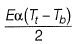(b)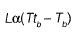(c)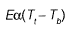(d)  Zero
(d)
Solution: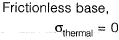Question 5.  Given the following data: design life n = 15 years, lane distribution factor D = 0.75, annual rate of growth of commercial vehicles r = 6%, vehicle damage factor P= 4 and initial traffic in the year of completion of construction = 3000 Commercial Vehicles Per Day (CVPD). As per IRC : 37-2012, the design traffic in terms of cumulative number of standard axles (in million standard axles, up to two decimal places) is ________     [2018 : 2 Marks, Set-I]
Solution: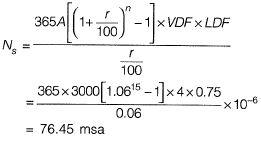Question 6. The radii of relative stiffness of the rigid pavements P and Q are denoted by lp and lQ, respectively. The geometric and material properties of the concrete slab and underlying soil are given below: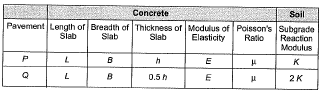The ratio (up to one decimal place) of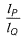is _______.
[2017 : 2 Marks, Set-II]
Solution:

Relative stiffness,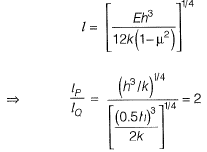Question 7.  In the context of the IRC 58-2011 guidelines for rigid pavement design, consider the following pair of statements.
I. Radius of relative stiffness is directly related to modulus of elasticity of concrete and inversely related to Poisson’s ratio.
II. Radius of relative stiffness is directly related to thickness of slab and modulus of subgrade reaction.
Which one of the following combinations is correct?   [2016 : 1 Mark, Set-II]
(a) I. True; II. True
(b) I. False; II. False
(c) I. True; II. False
(d) I. False; II. True
(b)
Solution: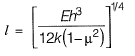As μ increases then 1 - μ2 decreases
∴ Relative stiffness also increases.
∴ Statement 1 is false.
As modulus of subgrade reaction (k) increases, (I) decreases
∴ Statement 2 is false.

Question 8.  A traffic survey conducted on a road yields an average daily traffic count of 5000 vehicles. The axle load distribution on the same road is given in the following table: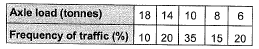The design period of the road is 15 years, the yearly traffic growth rate is 7.5% and the load safety factor (LSF) is 1.3. If the vehicle damage factor (VDF) is calculated from the above data the design traffic (in million standard axle load, MSA) i s _________ .     [2014 : 2 Marks, Set-I]

Solution: Vehicle damage factor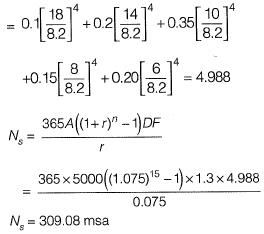Question 9. The following statements are related to temperature stresses developed in concrete pavement slabs with free edges (without any restraint):
P. The temperature stresses will be zero during both day and night times if the pavement slab is considered weightless.
Q. The temperature stresses will be compressive at the bottom of the slab during night time if the self-weight of the pavement slab is considered.
R. The temperature stresses will be compressive at the bottom of the slab during day time if the self-weight of the pavement slab is considered
The TRUE statement(s) is(are)     [2014 : 1 Mark, Set-I]
(a) P only
(b) Q only
(c) P and Q
(d) P and P only
(c)
Solution:
The temperature stress will be tensile at the bottom of the slab during the day time if the self weight of the pavement slab is considered.

Question 10. Select the strength parameter of concrete used in design of plain jointed cement pavement from the following choices:    [2013 : 1 Mark]
(a) Tensile strength
(b) Compressive strength
(c) Flexural strength
(d) Shear strength
(c)
Solution:
Rigid pavement transfers the load by bending action, so flexural strength of concrete used should be high.

Question 11. A pavement designer has arrived at a design traffic of 100 million standard axles for a newly developing national highway as per IRC:37 guidelines using the following data : life = 15 years, commercial vehicle count before pavement construction = 4500 vehicles/day, annual traffic growth rate = 8%. The vehicle damage factor used in the calculation was     [2012 : 2 Marks]
(a) 1.53
(b) 2.24
(c) 3.66
(d) 4.14

Solution:
Number of commercial vehicles per day,
A = 4500
Annual traffic growth rate,
r = 8%
Design life,
n = 15 years
The cumulative standard axles,
N8 = 100 x 106
Vehicle damage factor used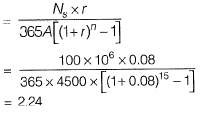Question 12. Consider the following statements in the context of cement concrete pavements:
1. Warping stresses in cement concrete pavements are caused by the seasonal variation in temperature.
2. Tie bars are generally provided across transverse joints of cement concrete pavements.
The correct option evaluating the above statements is    [2010 : 2 Marks]
(a) 1 true and 2 false
(b) 1 false and 2 true
(c) 1 true and 2 true
(d) 1 false and 2 false
(d)
Solution:
Warping stresses in cement concrete pavements are caused by the daily variation in temperature. Tie bars are generally provided across longitudinal joints of cement concrete pavements

Offer running on EduRev: Apply code STAYHOME200 to get INR 200 off on our premium plan EduRev Infinity!

,

,

,

,

,

,

,

,

,

,

,

,

,

,

,

,

,

,

,

,

,

;